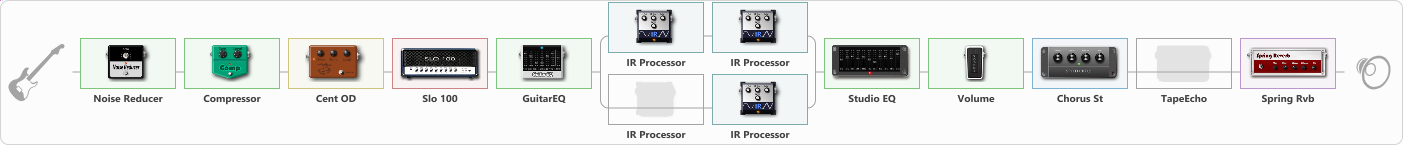# Justice/Death Magnetic Test (Metallica tone test)

Discussion in 'ToneLib-GFX presets' started by clever1, Apr 28, 2021.

1. Justice/Death Magnetic Test (Metallica tone test)

Preset name: Justice/Death Magnetic G12T-75 test

Just a test with the cabs used to record ...And Justice For All (RTL maybe and also MoP for sure) and an IR from AFJA and also a Death Magnetic IR. I think that the Justice IR makes it sound not that good but the DM IR levels it a little bit so I left it like this.

Effects chain:Effect: "Noise Reducer" (Dynamics / Filter), active - "yes"
{
"Sens" = 49
"Mode" = Hard
}

Effect: "Compressor" (Dynamics / Filter), active - "yes"
{
"Sense" = 40
"Level" = 38
}

Effect: "Cent OD" (Overdrive / Distortion), active - "yes"
{
"Drive" = 16
"Tone" = 41
"Level" = 44
}

Effect: "Slo 100" (Amp simulators), active - "yes"
{
"Gain" = 82
"Bass" = 74
"Middle" = 10
"Treble" = 46
"Presence" = 63
"Master" = 100
"Level (dB)" = 2
}

Effect: "GuitarEQ" (Dynamics / Filter), active - "yes"
{
"160 Hz" = 3
"400 Hz" = 1
"800 Hz" = 3
"1.6 kHz" = 5
"3.2 kHz" = 1
"6.4 kHz" = 5
"12 kHz" = 0
"Level (dB)" = 3
}

Effect: "Splitter" (Dynamics / Filter)
{
"A-Bypass" = Off
"A-Pan" = 54
"A-Level" = 56
"B-Bypass" = Off
"B-Pan" = -26
"B-Level" = 64

'A' branch:
{

Effect: "IR Processor" (Cabinets), active - "yes"
{
"IR" = death_magnetic_IR
"Low Cut (Hz)" = 0
"Hi Cut (kHz)" = 20.0
"Mix" = 32
"Level (dB)" = 0
}

Effect: "IR Processor" (Cabinets), active - "yes"
{
"IR" = OD-UK112-T75-CND-AU-1-P00-50
"Low Cut (Hz)" = 0
"Hi Cut (kHz)" = 17.0
"Mix" = 100
"Level (dB)" = 1
}
}
'B' branch:
{

Effect: "IR Processor" (Cabinets), active - "no"
{
"IR" = justice_LR_Stereo_IR
"Low Cut (Hz)" = 0
"Hi Cut (kHz)" = 20.0
"Mix" = 40
"Level (dB)" = 0
}

Effect: "IR Processor" (Cabinets), active - "yes"
{
"IR" = OD-UK112-T75-DYN-US-6-P00-20
"Low Cut (Hz)" = 0
"Hi Cut (kHz)" = 20.0
"Mix" = 100
"Level (dB)" = 2
}
}
}

Effect: "Studio EQ" (Dynamics / Filter), active - "yes"
{
"31 Hz" = 0
"62 Hz" = 0
"125 Hz" = 0
"250 Hz" = 0
"500 Hz" = 0
"1 kHz" = 1
"2 kHz" = 1
"4 kHz" = 3
"8 kHz" = 2
"16 kHz" = 0
"Level (dB)" = 0
}

Effect: "Volume" (Dynamics / Filter), active - "yes"
{
"Pedal Pos." = 33
"Pan" = 0
}

Effect: "Chorus St" (Modulation / Sfx), active - "yes"
{
"Speed" = 1.9
"Depth" = 26
"Center" = 3.9
"Mix" = 27
}

Effect: "TapeEcho" (Delay), active - "no"
{
"Time" = 419
"Feedback" = 22
"Tone" = 54
"LoDamp" = 19
"Mix" = 57
}

Effect: "Spring Rvb" (Reverberation), active - "yes"
{
"Time" = 4.1
"PreDelay" = 0
"LoDamp" = 16
"HiDamp" = 23
"Mix" = 30
}

Note: You will need to download and install the ToneLib-GFX software to use the preset.

File size:
7.5 KB
Views:
2,728